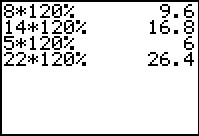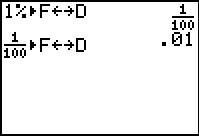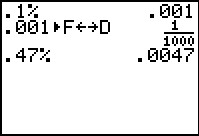# Activities

••• ##### Subject Area

• Math: Middle Grades Math: Number Sense

• ##### Author6-8

45 Minutes

• ##### Device
• TI-73 Explorer™
• TI-Navigator™
• ##### Software

LearningCheck™ Creator
TI Connect™

## Percents - Small and Large#### Activity Overview

In this activity, students will explore percents greater than 100% and less than 1%. Students will use a 100 grid to represent percents and convert between fractions, decimals, and percents.

#### Key Steps

•In this activity, students will explore percents greater than 100%. They start by calculating prices for a fund raiser using the percent key on the Home screen of the calculator.

•Students then convert percents to fractions and decimals for comparison using the Fraction-Decimal equivalence key and by shading a 100-grid.

•Finally, students look at percents less than 1%. In the TI-NavigatorTM extension, students use a number line to assess their understanding of percents.